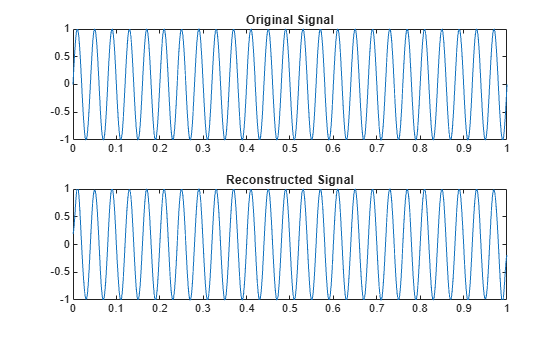# Discrete Cosine Transform

The discrete cosine transform (DCT) is closely related to the discrete Fourier transform (DFT). The DFT is actually one step in the computation of the DCT for a sequence. The DCT, however, has better energy compaction than the DFT, with just a few of the transform coefficients representing the majority of the energy in the sequence. This property of the DCT makes it useful in applications such as data communications and signal coding.

### DCT Variants

The DCT has four standard variants. For a signal x of length N, and with ${\delta }_{k\ell }$ the Kronecker delta, the transforms are defined by:

• DCT-1:

`$y\left(k\right)=\sqrt{\frac{2}{N-1}}\sum _{n=1}^{N}x\left(n\right)\frac{1}{\sqrt{1+{\delta }_{n1}+{\delta }_{nN}}}\frac{1}{\sqrt{1+{\delta }_{k1}+{\delta }_{kN}}}\mathrm{cos}\left(\frac{\pi }{N-1}\left(n-1\right)\left(k-1\right)\right)$`

• DCT-2:

`$y\left(k\right)=\sqrt{\frac{2}{N}}\sum _{n=1}^{N}x\left(n\right)\frac{1}{\sqrt{1+{\delta }_{k1}}}\mathrm{cos}\left(\frac{\pi }{2N}\left(2n-1\right)\left(k-1\right)\right)$`

• DCT-3:

`$y\left(k\right)=\sqrt{\frac{2}{N}}\sum _{n=1}^{N}x\left(n\right)\frac{1}{\sqrt{1+{\delta }_{n1}}}\mathrm{cos}\left(\frac{\pi }{2N}\left(n-1\right)\left(2k-1\right)\right)$`

• DCT-4:

`$y\left(k\right)=\sqrt{\frac{2}{N}}\sum _{n=1}^{N}x\left(n\right)\mathrm{cos}\left(\frac{\pi }{4N}\left(2n-1\right)\left(2k-1\right)\right)$`

The Signal Processing Toolbox function `dct` computes the unitary DCT of an input array.

### Inverse DCT Variants

All variants of the DCT are unitary (or, equivalently, orthogonal): To find their inverses, switch k and n in each definition. DCT-1 and DCT-4 are their own inverses. DCT-2 and DCT-3 are inverses of each other:

• Inverse of DCT-1:

`$x\left(n\right)=\sqrt{\frac{2}{N-1}}\sum _{k=1}^{N}y\left(k\right)\frac{1}{\sqrt{1+{\delta }_{k1}+{\delta }_{kN}}}\frac{1}{\sqrt{1+{\delta }_{n1}+{\delta }_{nN}}}\mathrm{cos}\left(\frac{\pi }{N-1}\left(k-1\right)\left(n-1\right)\right)$`

• Inverse of DCT-2:

`$x\left(n\right)=\sqrt{\frac{2}{N}}\sum _{k=1}^{N}y\left(k\right)\frac{1}{\sqrt{1+{\delta }_{k1}}}\mathrm{cos}\left(\frac{\pi }{2N}\left(k-1\right)\left(2n-1\right)\right)$`

• Inverse of DCT-3:

`$x\left(n\right)=\sqrt{\frac{2}{N}}\sum _{k=1}^{N}y\left(k\right)\frac{1}{\sqrt{1+{\delta }_{n1}}}\mathrm{cos}\left(\frac{\pi }{2N}\left(2k-1\right)\left(n-1\right)\right)$`

• Inverse of DCT-4:

`$x\left(n\right)=\sqrt{\frac{2}{N}}\sum _{k=1}^{N}y\left(k\right)\mathrm{cos}\left(\frac{\pi }{4N}\left(2k-1\right)\left(2n-1\right)\right)$`

The function `idct` computes the inverse DCT for an input sequence, reconstructing a signal from a complete or partial set of DCT coefficients.

### Signal Reconstruction Using DCT

Because of the energy compaction property of the DCT, you can reconstruct a signal from only a fraction of its DCT coefficients. For example, generate a 25 Hz sinusoidal sequence sampled at 1000 Hz.

```t = 0:1/1000:1; x = sin(2*pi*25*t);```

Compute the DCT of this sequence and reconstruct the signal using only those components with value greater than 0.1. Determine how many coefficients out of the original 1000 satisfy the requirement.

```y = dct(x); y2 = find(abs(y) < 0.1); y(y2) = zeros(size(y2)); z = idct(y); howmany = length(find(y))```
```howmany = 64 ```

Plot the original and reconstructed sequences.

```subplot(2,1,1) plot(t,x) ax = axis; title('Original Signal') subplot(2,1,2) plot(t,z) axis(ax) title('Reconstructed Signal')```One measure of the accuracy of the reconstruction is the norm of the difference between the original and reconstructed signals, divided by the norm of the original signal. Compute this estimate and express it as a percentage.

`norm(x-z)/norm(x)*100`
```ans = 1.9437 ```

The reconstructed signal retains approximately 98% of the energy in the original signal.